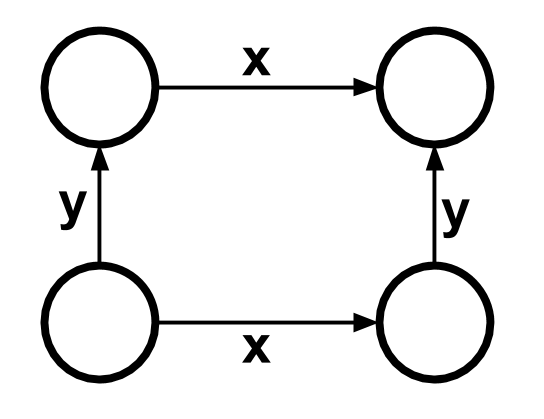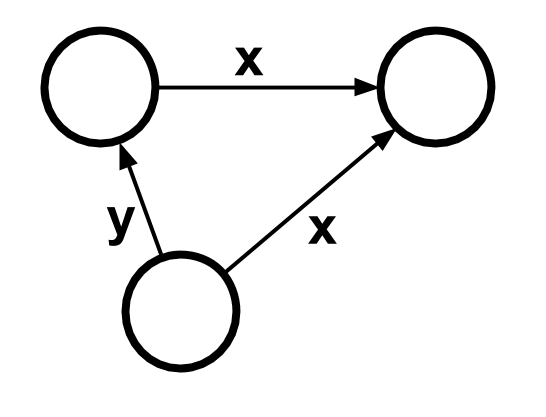Testing is tedious! At least that's what I thought before I stumbled over property-based testing – a simple approach that allows you to write fewer tests yet gain more confidence in your code.

In traditional testing, you define concrete inputs and test whether they result in the desired output. In property-based testing, you define certain conditions that are always true for any input (those are called properties). In mathematics, there's the ∀ operator for that. In Dart, now there's Glados.Here are some benefits:

• Write fewer tests. Let Glados figure out inputs that break your properties.
• 🌌 Test for all possible inputs. Well, not all. But a wide variety.
• 🐜 Get a concise error report. Glados simplifies inputs that break your tests.
• 🤯 Understand the problem domain better by thinking of properties.

## Quickstart

``````dev_dependencies:
test: ...
``````

Use `Glados<...>().test(...)` instead of the traditional `test(...)`.

``````// Running this test shows you that it fails for input 21.
expect(a * 2, lessThan(42));
});
``````

You can test with multiple inputs.

``````Glados2<String, int>().test((a, b) { ... });
``````

Instead of using type parameters, you can customize inputs using `any`.

``````Glados(any.lowercaseLetter).test((letter) { ... });
``````

Do you want to test with your data classes? Here's how.

You can also customize the size of the generated inputs.

## Comprehensive example

Suppose you write a function that tries to find the maximum in a list. I know – that's pretty basic – but it's enough to get you started. Here's a wrong implementation:

``````/// If the list is empty, return null, otherwise the biggest number.
int? max(List<int> input) => null;
``````

To be sure that the function does the right thing, you might want to write some tests. Here's how those would look like in traditional unit testing:

``````test('maximum of empty list', () {
expect(max([]), equals(null));
});
test('maximum of non-empty list', () {
expect(max([40, 2, 10]), equals(40));
});
``````

Not familiar with the syntax of the `test` package? Here are the docs.

Executing `pub run test path/to/tests.dart` should show that the second test fails.

In property-based testing, you look for properties – conditions that should be true for any input. For example, if `max` produces `null`, the list should be empty:

``````Glados<List<int>>().test('maximum is only null if the list is empty', (list) {
if (max(list) == null) {
expect(list, isEmpty);
}
});
``````

Just create a `Glados` instance and call its `test` method instead of using the normal `test` function. `Glados` takes a generic type parameter – in this case, `List<int>`. It then tests your code with a variety of inputs of that type. All of them need to succeed for the whole test to succeed.

Running the test should produce something like this:

``````Tested 1 input, shrunk 25 times.
Failing for input: 
...
``````

Glados discovered that a list with some content breaks the condition!

Let's modify our `max` function to pass this test:

``````int? max(List<int> input) => 42;
``````

We need to add another property test to reject this function as well. Arguably the most prominent property for `max` is the following: The maximum should be greater than or equal to all items of the list:

``````Glados(any.nonEmptyList(any.int)).test('maximum is >= all items', (list) {
var maximum = max(list);
for (var item in list) {
expect(maximum, greaterThanOrEqualTo(item));
}
});
``````

Instead of defining type parameters, you can also pass in generators to Glados to customize which values it generates. You can find all available generators as fields on the `any` value. In this case, we only test with non-empty lists because we handled the empty list in the first test.

Running the tests produces the following result:

``````Tested 35 inputs, shrunk 117 times.
Failing for input: 
...
``````

Glados detected that the property breaks if the input list contains a `43`.

Let's add a more practical implementation for `max`:

``````int max(List<int> input) {
if (input.isEmpty) {
return null;
}
var max = 0;
for (var item in input) {
if (item > max) {
max = item;
}
}
return max;
}
``````

That change fixes the tests but still doesn't work for lists containing only negative values. So, let's add a final test:

``````Glados(any.nonEmptyList(any.int)).test('maximum is in the list', (list) {
expect(list, contains(max(list)));
});
``````

I'll leave implementing the function correctly to you, the reader.

But whatever solution you come up with, it'll be correct: Our tests aren't merely some arbitrary examples anymore. Instead, they correspond to the actual mathematical definition of max.

## How to find properties

Finding properties can seem difficult at times, but you'll get better at finding them, and you can start with obvious ones first. They don't have to be perfect – testing a few simple properties can still be better than traditional unit tests.

To get you started, here are a bunch of patterns that can help you to find properties:

Commutativity: The order of the operations doesn't matter.
• `list.reversed.map(...) == list.map(...).reversed`
• a + b = b + aTransform: Certain operations don't affect the result of another operation.
• After reversing or shuffling a list, the maximum should stay the same.Inverse: Sometimes, you can invert operations.
• Reversing a list twice
• Adding and subtracting the same value
• Serializing and deserializing an objectIdempotence: Certain operations don't change the value if they're applied a second time.
• Sorting a list twice
• Running a code formatter on a source code twiceOracle: If you have a correct implementation and want to test a more efficient one, just compare the results.
• Quicksorting a list is the same as bubblesorting it## How does it work?

• 🌍 The exploration phase: Glados generates increasingly complex, random inputs until one breaks the property or Glados reaches the maximum number of runs.
• 🐜 The shrinking phase: This phase only happens if Glados found an input that breaks the property. In this case, it gradually simplifies the input and returns the smallest input that's still breaking the property.

Generators are responsible for generating values. A `Generator<T>` is a function that takes a `random` and `size` and produces a `Shrinkable<T>`. The `random` parameter should be used as the only source of randomness to guarantee reproducibility when running tests multiple times. The `size` parameter indicates a rough estimate of how complex the returned value should be. For example, the generator for `int` produces `int`s in the range from `-size` to `size`.

A `Shrinkable<T>` is a wrapper around a `T` (it has a `value` getter for that). It also has a `shrink` method, which produces an `Iterable` of `Shrinkable<T>` values similar to the current value but smaller. Smaller means that if you would call `shrink` repeatedly on the smaller values and their children, grandchildren, etc., the program should eventually terminate (aka the transitive hull concerning `shrink` should be finite and acyclic).

The basic types all have corresponding generators implemented. You can find all generators on `any`.

## How to write generators

For simple data classes with some fields, this is how the generator might look like:

``````class User {
final String name;
final int age;
}

extension AnyUser on Any {
Generator<User> get user => combine2(any.string, any.int, (name, age) {
return User(name, age);
});
}
``````

For enums, this is how the generator might look like:

``````enum Ripeness { ripe, unripe }

extension AnyRipeness on Any {
Generator<Ripeness> get ripeness => choose(Ripeness.values);
}
``````

If you want to customize generators further, like only generate valid email addresses, you might need to work on a lower level of abstraction. Just check out the source code of existing generators for some examples.

Here's how to set a generator as the default generator for a type:

``````Any.setDefault<String>(any.emailAddress);

// Uses the any.emailAddress generator based on the type parameters.
``````

## Customizing the exploration phase

You can configure the exploration phase by customizing the `ExploreConfig`.

For example, if you want to test some code with massive inputs, you might adjust `ExploreConfig`'s parameters so that Glados starts with already massive inputs and generates much bigger inputs after just a few runs:

``````Glados(any.email, ExploreConfig(
initialSize: 100, // Start quite big
speed: 10,        // and increase the input size by 10 each run,
numRuns: 10,      // but only do 10 runs instead of 100.
)).test('my test', (input) {
...
});
``````

`ExploreConfig` also has a `random` parameter, which you can provide with a custom `Random` instance. By default, `ExploreConfig` uses a `Random` instance created with a fixed seed so that your tests are deterministic.

## What's up with the name?

GLaDOS is a charming robot in the Portal game series. She's the head of the Aperture Science Laboratory facilities, where she spends the rest of her days testing. So I thought that's quite a fitting name. 🥔

By the way, both Portal games are great. If you haven't played them, definitely check them out.

🍰